# Project Euler Problem 15 in F#

With Project Euler Problem 15 we approach the fascinating world of graphs (but, as we shall see, only in a very tangential fashion):

Starting in the top left corner of a 2x2 grid, there are 6 routes (without backtracking) to the bottom right corner.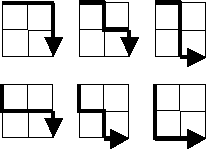How many routes are there through a 20x20 grid?

## Analysis

With pen and paper the fact that a 2x2 grid has 6 possible routes can be easily confirmed. However, as soon as the grid size grows, it's simply not practical to start drawing all the possible ways to reach the lowest right corner from the top left one.

In order to find a general solution, it helps trying a dynamic programming approach: we first try to solve a smaller problem and then combine the solutions of more sub-problems to find the overall answer.

The smallest problem that we can consider is the number of possible routes in a 1x1 grid. What could be simpler? Considering all the three possible starting corners of such a grid we can see that:

• From both the bottom-left and the top-right corner there's only one possible route to the destination.
• From the top-left corner there are trivially two possible routes.

In the picture below, I have marked for each corners the number of possible routes to the destination for the 1x1 grid: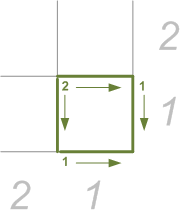We can now consider the bigger 2x2 grid enclosing the smaller 1x1 grid representing the sub-problem we solved earlier:

• Both points on the outer sides of the grid have only one possible route to the destination. In fact, from there you can only proceed along the edge. So, we mark both of these points with 1.
• Let's now consider the point immediately above the bottom left one on the grid: from this point we can either move down (to the point that we just marked with a 1 or right to the point of the 1x1 grid that we had marked with a 2 previously. Hence, from this point we have 1 possible route if we decide to go down or 2 possible routes if we decide to go right: that makes 3 possible routes that can lead from this point to the destination.

We can generalize and deduce that for each point in the grid, the number of possible routes to the destination is equal to the sum of the routes available from the point immediately down and the point immediately right (points on the outer edge having only 1 possible route). This is the key to solve this problem for any kind of grid.

So, we have informally demonstrated the result for the 2x2 grid: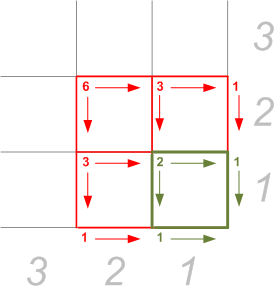And similarly we can resolve the problem for a 3x3 grid: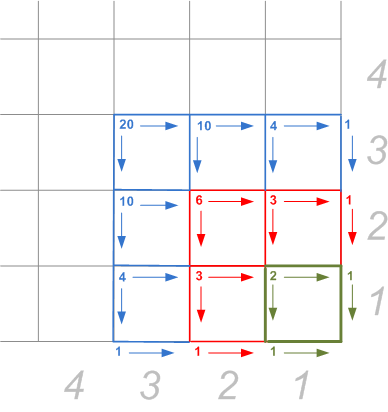Now, if you look closely at the patterns of the possible routes for each point in the grid, you may recognize what in Italy we call Il triangolo di Tartaglia (Tartaglia's Triangle), which everywhere else in the world is most probably known as Pascal's Triangle: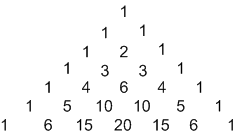(note that the triangle obviously continues ad infinitum).

The solution for a square grid of any size lies in the vertical diagonal (if you excuse the quasi-oxymoron), that is 1, 2, 6, 20, etc... If we start counting rows from the top, first row being row 0, second row being row 1 and so on, and columns from the left also starting from 0, we can see that for a square grid of size n, the number of routes is given by the item in the n column within the 2*n row.

Now, it would be trivial, if somehow boring, to solve the problem for a 20x20 grid using a spreadsheet. All you have to do is to create the 20 x 2 = 40 rows of the Pascal triangle ( with some copy and paste of the right formula for each cell), and pick the 20th element from that road.

However, you don't actually have to create the whole triangle: the values of each row can be calculated using they binomial expansion: for a given row r, the value at column c is given by the following formula:

$$v(c) = \binom{r}{c} = \frac{r}{c!(r-c)!}$$

Perhaps more interestingly, you can calculate the same value without resorting to the factorial by using the following recursive formula (where r' is the row number +1 and  c is the column index):

\begin{align*} v(c) &= v(c-1) \frac{r'-c}{c} \\ v(0) &= 1 \end{align*}

## Solution

So, after all this analysis, the solution comes up to be quite simple and maybe not that interesting per se. The recursive formula to calculate the values from the triangle's rows fits naturally the F# pattern matching:

Updated: